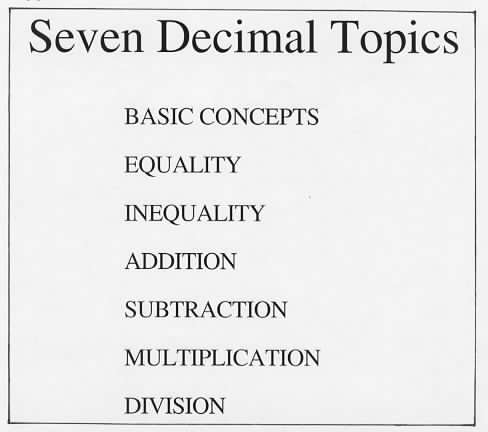Home
 Introduction to Decimal Squares (nonthreatening visual models) . Materials: Deck of Decimal Squares for each group or pair of people.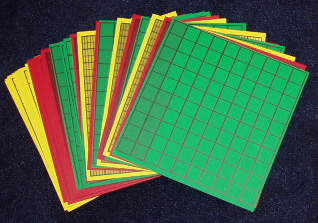. Ask each group to unbundle the decks of Decimal Squares and to list several observations. Here are a few of the many comments that are possible: the red squares are divided into 10 equal parts; the green squares into 100 equal parts and the yellow squares into 1000 equal parts; there is one of each red square with from 1 to 9 shaded parts the shading on the green squares increases by 5 parts from square to square and the shading on the yellow squares increases by either 25 or 50 parts from square to square. Discuss the full deck by asking the groups to determine the number of each type of square: 9 red green and 29 yellow. If it does not come up note that these squares can be described without using decimals. For example place the green transparent square for .35 on the overhead and note that it can be described as a green square with 35 parts shaded or a square with 35 shaded parts out of 100 etc.. Ask each group to select a square and write a description of it without using decimals. Note that such verbal descriptions of visual models are nonthreatening and will help to establish an understanding of decimal symbolism.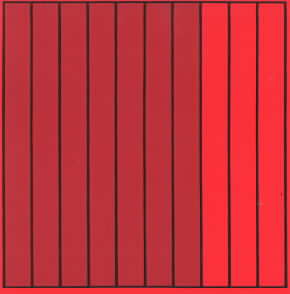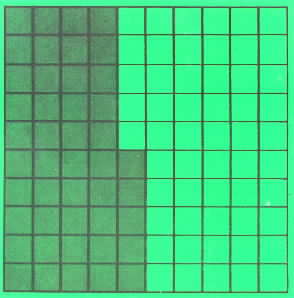red square with 7 parts shaded 7 shaded parts out of 10 green square with 45 parts shaded 45 shaded parts out of 100 yellow square with 275 parts shaded 275 shaded parts out of 1000 The students in this activity are verbally describing the squares they want from other players with the objective of obtaining two or more shaded squares whose total shaded amount is one whole square.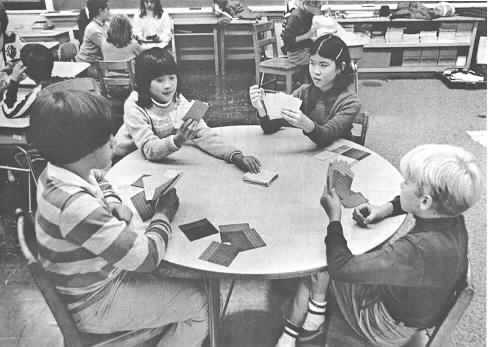Photo courtesy of Herb Moyer Seven Decimal Topics Show the following transparency of 7 decimal topics. Point out that each of these decimal topics will be introduced through games and activities to provide understanding of decimal symbolism before using rules for operations with decimals.